# 1st PUC Chemistry Previous Year Question Paper March 2019 (South)

Students can Download 1st PUC Chemistry Previous Year Question Paper March 2019 (South), Karnataka 1st PUC Chemistry Model Question Papers with Answers helps you to revise the complete Karnataka State Board Syllabus and score more marks in your examinations.

## Karnataka 1st PUC Chemistry Previous Year Question Paper March 2019 (South)

Time: 3.15 Hours
Max Marks: 70

Instruction:

1. The questions paper has five parts A, B, C, D and E. All parts are compulsory.
2. Write balanced chemical equations and draw labeled diagram wherever allowed.
3. Use log tables and simple calculations f necessary (use of scientific calculations is not allowed).

Part – A

I. Answer ALL of the following (each question carries one mark): ( 10 × 1 = 10 )

Question 1.
What is the SI unit of density?
kgm-3

Question 2.
Define critical temperature.
Temperature above which a gas cannot be liquified by applying pressure.

Question 3.
Define pH of a solution.
Negative logarithm to base ten of the hydrogen ion concentration is called pH.

Question 4.
State modern periodic law.
Properties of elements are periodic functions of their atomic number.Question 5.
What is the oxidation number of Mn in KMnO4.
+ 7

Question 6.
Write the general-electronic configuration of alkali metals.
nS1

Question 7.
What is dry ice.
Solid CO2.

Question 8.
Write the composition of water gas.
CO + H2

Question 9.
Explain the homolytic fission.
Symmetrical cleavage of covalent bond.

Question 10.
Name the catalyst used in Friedel-craft reaction.
Anhydrous AlCl3Part – B

II. Answer FIVE of the following questions carrying TWO marks: ( 5 × 2 = 10 )

Question 11.
Calculate the molarity of a solution containing 2.3 moles of solute dissolved in 4.6 litres.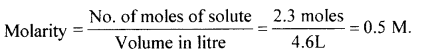Question 12.
State Boyle’s law and give its mathematical form.
At constant temperature, volume of a given fixed of a mass is inversely proportional to its pressure.
PV = constant

Question 13.
The dipole moment in BF3 is zero. Explain.
In BF3 there are 3 dipole. Its dipole moment is zero. This is possible only when the molecule is symmetric, BF3 has trigonal planar shape.
The bond angle is 120°. The resultant m of any two dipoles is equal and opposite to the third dipole. So the net dipole moment is zero.

Question 14.
Give the important uses of plaster of Paris.

1. In surgery for setting
2. In making blackboard chalks.Question 15.
Write the resonance structure of carbonate ion (CO3-3).Question 16.
Explain Kolbe’s reaction of preparation of ethane.
A concentrated sodium salt of a carboxylic acid is electrolysed. The alkane is formed at the anode as one of the products. This process is called Kolbe’s electrolysis.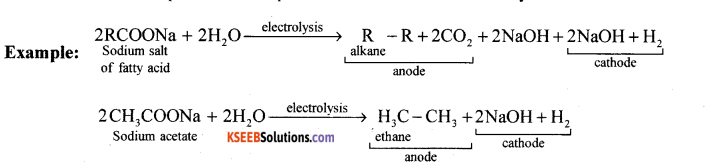Question 17.
Explain the nitration of benzene.
Benzene on treating with nitrating mixture, nitrobenzene is formed.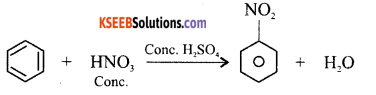Question 18.
Name the components of photochemical smog.
Nitrogen oxides, ozone, organic derivatives.

Part – C

III. Answer any FIVE of the following questions carrying THREE marks: ( 5 × 3 = 15 )

Question 19.
Define ionisation enthalpy. How it varies along a period and down the group.
The amount of energy required to remove the most loosely bound electron from an isolated gaseous atom. Ionization energy increases along the period and decreases down the group.Question 20.
Explain sp3 hybridisation by taking methane as example.
CH4 – Methane Molecule. The Molecular formula of Methane is CH4
Electronic configuration of C is ground state – 1s22s22p2
Electronic configuration of C in excited state – 1s2 2s1 2p3
Valance orbital representation –The valence orbital contains unpaired electron. Elence sp3 hybridized carbon combine with 4 hydrogen atom forms methane molecule.

Question 21.
Write the electronic configuration of Hydrogen molecule. Calculate its bond order and mention its magnetic property.
Electronic configuration – σ1S 2
Bond order = $$\frac{2-0}{2}$$ = 1.
Diamagnetic property.

Question 22.
Write the three postulates of VSEPR Theory.

1. Bonding : The bond formation takes place if there exists impaired electron in the valance shell.
2. Shape : The geometry or the shape of a molecule depends on the number of valence shell electrons surroundings the central atom.
3. Repulsion : Electron pair tend to repell one another because electron clouds have similar charge.
4. Stability : As a result of electron pair repulsion these electron pairs try to stay apart as possible in order to attain minimum energy and maximum stability.
5. Repulsive interaction : Lane pair with lane pair electrons are having maximum repulsive interaction, bond pair – bond pair electrons are having minimum repulsive interaction. When angle of repulsion decreases angle between the electron pair increases.
(109°. 28′ less repulsion, 104° more repulsion).

Question 23.
Balance the following redox reaction by using oxidation number method.
MnO2 + Br→ Mn+2 + Br2 + H2O (acid medium)Question 24.
(a) Explain ionic hydrides with an example.
These contain positive metal ions and negative hydride ions. These are also called saline hydrides. Ex: (Na+H ) sodium hydride, [Ca2+ (H)2] calcium hydride.

(b) What is temporary hardness of water?
Water containing bicarbonates of calcium and magnesium.Question 25.
(a) Explain the diagonal relationship between Lithium and Magnesium.
(a) Both Li and Mg are hard, (b) Li(OH) and Mg(OH)2 are bases, (c) Both Li and Mg react with atmospheric nitrogen on heating gives their nitrides, (d) Both Li and Mg forms only monoxide but not peroxides and superoxides.

Question 26.
Explain the structure of diborane.

1. The four terminal hydrogen atoms and two boron atoms lie in one plane.
2. Two bridging hydrogen atom lie above and below the plane.
3. Bonding between boron atom and terminal hydrogen atom is normal covalent bond whereas with bridging hydrogen atom it is 3 centred 2 electron bond.
4. Banana bond is formed with bridging H atoms.

Part – D

IV. Answer any FIVE of the following questions carrying FIVE marks: ( 5 × 5 = 25 )

Question 27.
(a) A compound contains 4.07% Hydrogen, 24.47% Carbon and 71.65% Chlorine.
Its molar mass is 98.96g. What are its empirical formula and molecular formula.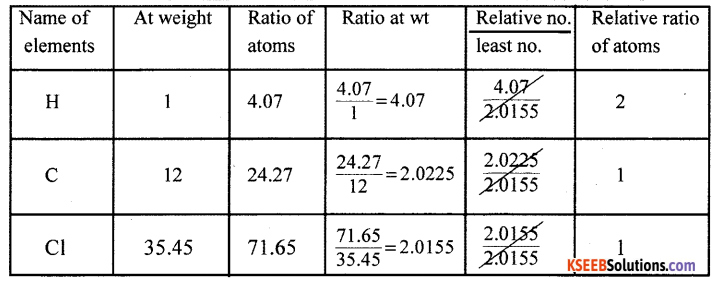Empirical Formula H2C1Cl

(b) Calculate the molecular mass of glucose.
C6H12O6 – 180 gmol-1

Question 28.
(a) Give the postulates of Bohr’s theory of an atom.
Bohr’s model of an atom, the postulates are:

1. Electrons revolve around the nucleus of an atom in a certain definite path called orbit or stationary state of shell.
2. The shells are having different energy levels denoted as K, L, M, N…
3. As long as the electron remains in an orbit, they neither absorb nor emit energy.
4. The electron can move only in that orbit in which angular momentum is quantized,
i.e., the angular momentum of the electron is an integral multiple of $$\frac{h}{2 \pi}$$

(b) Write down the value of n, 1 and m for electron present in 2Pz orbital.
n = 2, 1 = 1, m = -1,0,+l.

Question 29.
(a) Explain the significance of quantum numbers.
(i) Principal Quantum number : Determines energy and size of the orbital.
(n)
(ii) Azimuthal Quantum number : Determines the shape of the orbitals (sub-shells).
(l)
(iii) Magnetic Quantum number : Determines the orientation of the orbitals.
(m)
(iv) Spin Quantum number : Determines the spin motion of the orbitals.
(s)(b) State the Hund’s rule of maximum multiplicity.
Electron pairing does not take place until orbitals of same energy are singly occupied.

Question 30.
(a) Write any three postulates of kinetic theory of gases.

1. Gases are made up of large number of the minute particles.
2. Pressure is exerted by a gas.
3. There is no loss of kinetic theory.
4. Molecules of gas attract on one another.
5. Kinetic energy of the molecule is directly proportional to absolute temperature.
6. Actual volume of the gaseous molecule is very small.
7. Gaseous molecules are always in motion.
8. There is more influence of gravity in the movement of gaseous molecule.

(b) Write Ideal gas equation and explain its terms.
PV = nRT
P = Pressure, n = No. of moles, V = Volume,
R – Gas Constant, T = Temperature

Question 31.
(a) Calculate the standard enthalpy of formation of Benzene. Given that the enthalpies of combustion of Carbon, Hydrogen and Benzene are -393.5 KJ mol-1, -285.83 KJ mol-1 and -3267 KJ mol-1 respectively.(b) State second law of thermodynamics.
“The net entropy of the universe is continuously increasing” (or) “total entropy of the system
and the surroundings increases in any spontaneous process i.e., ASsys(em + ASsurround; > 0 , for any sponteneous process.”

Question 32.
(a) What is an intensive property? Give an example.
A property which is independent on the quantity of matter present in the system.
Example: Density’, Temperature.

(b) State Hess’s law of constant heat summation.
Hess’s law : Whether a chemical reaction takes place in a single step or in several steps, the ‘ total change in enthalpy remains the same.
Consider the formation of CO2 from C.

I Method (Single step): C(s) + O2 (g) → CO2(g); ∆H = -xkJ
Heat liberated = xkJ

II Method (Two step) : C(s) + $$\frac{1}{2}$$ O2 (g) → CO(g);∆H = -x,kJ
CO(g) +$$\frac{1}{2}$$ O2(g) → CO2(g) ; ∆H = -x2kJ .
Total heat liberated = (x1 + x2 )kJ
According to Hess’s law, x = x1 + x2

(c) What is exothermic reaction?
Reaction takes place with the liberation of heat.Question 33.
(a) To prove that pH + pOH = 14 at 298K.
The ionic product of water is given by [H] [OH] = Kw = 1.0 × 10-14 at 298 K
Taking log on both sides, log10[H+] + log10[OH] = log10 Kw = log10 1.0 × 10 -14
Multiplying by-ve sign, -log [H + ]-log10[OH ] = log10 Kw =-14.0000
wkt by definition, pH + pOH = pKw =14.

(b) Calculate the pH of 0.01M H2SO4 by assuming complete ionisation.
pH = -log [H+pH = -log [2 × 10-2 ]
[H+] = 0.02M
= 1.4990

(c) Define the Buffer action.
It is the ability or capacity of a buffer solution to resist the pH by addition of acid or base.

Question 34.
(a) Explain the common ion effect with an example.
Supperssion in the degree of dissociation of a weak electrolyte by the addition of a common ion is called common ion effect.

(b) Discuss amphoteric nature of water with an example.
A substance or molecule which acts both as an acid as well as base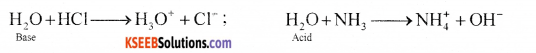(c) Mention the conjugate base of SO42-
HSO4-1

Part – E

V. Answer any TWO of the following questions carrying FIVE marks: ( 2 × 5 = 10 )

Question 35.
(a) With neat labelled diagram , describe the estimation of nitrogen by Dumas method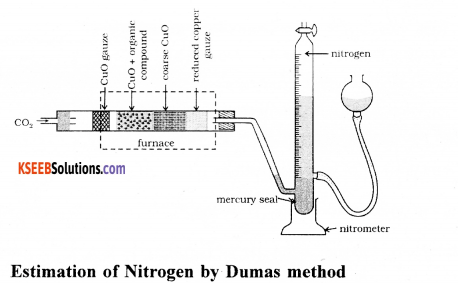Dumas method: The nitrogen containing organic compound, when heated with copper oxide in an atmosphere of carbon dioxide, yields free nitrogen in addition to carbon dioxide and water.
CxHyNz + (2x + y/2) CuO → x CO2 + y/2 H2O + z/2 N2 + (2x + y/2) Cu

Traces of nitrogen oxides formed, if any, are reduced to nitrogen by passing the gaseous mixture over a heated copper gauze. The mixture of gases so produced is collected over an aqueous solution of potassium hydroxide which absorbs carbon dioxide. Nitrogen is collected in the upper part of the graduated tube (Fig. 12.15).

Let the mass of organic compound = mg
Volume of nitrogen collected = V1mL
Room temperature = T1K
Volume of nitrogen at STP = $$\frac{\mathrm{p}_{1} \mathrm{V}_{1} \times 273}{760 \times \mathrm{T}_{1}}$$ (Let it be V mL)

Where p1 and V1 are the pressure and volume of nitrogen, p1 is different from the atmospheric pressure at which nitrogen gas is collected. The value of p1 is obtained by the relation;
p1 = Atmospheric pressure – Aqueous tension
22400 mL N2 at STP weighs 28 g.
V mL N2 at STP weighs = $$\frac{28 \times \mathrm{V}}{22400} \mathrm{g}$$
Percentage of nitrogen = $$\frac{28 \times V \times 100}{22400 \times m}$$

(b) Give any two differences between Inductive effect and Resonance effect.
Two or more compounds having same molecular formula but differ in the carbon chain are called chain isomers. This phenomenon is called chain isomerism. Ex: C4H10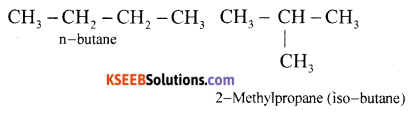Question 36.
(a) What are free radical? How are they formed?
A free radical can be defined as an atom or group of atoms having an odd or unpaired electron. Putting a dot (•) against the symbol of atom or group of atoms.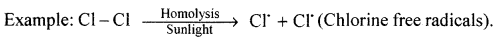(b) Write the structure of 3-methyl-pentanal.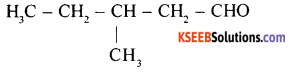(c) Give the example for +R effect.
-NH2, -OH,……..Question 37.
(a) Explain free radical mechanism of chlorination of m
In benzene, 6p electrons are present.
∴ n = 1 wkt Huckel’s rule is (4n + 2) p electrons.
∴ (4×1 + 2)π = 6π electrons.
Benzene is aromatic because it obeys Huckel rule and it is planar.

(b) Explain the mechanism of addition of HBr on propene.